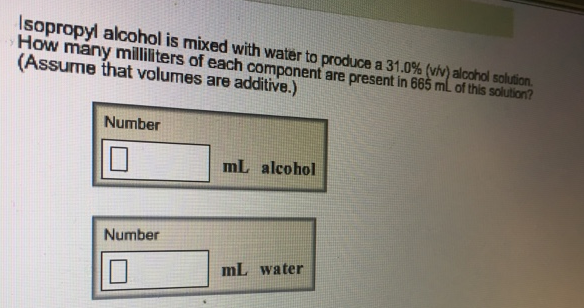# Problem: Isopropyl alcohol is mixed with water to produce a 31.0% (v/v) alcohol solution. How many milliliters of each component are present in 665 mL of this solution? (Assume that volumes are additive.)

###### FREE Expert Solution
85% (137 ratings)###### Problem Details

Isopropyl alcohol is mixed with water to produce a 31.0% (v/v) alcohol solution. How many milliliters of each component are present in 665 mL of this solution? (Assume that volumes are additive.)